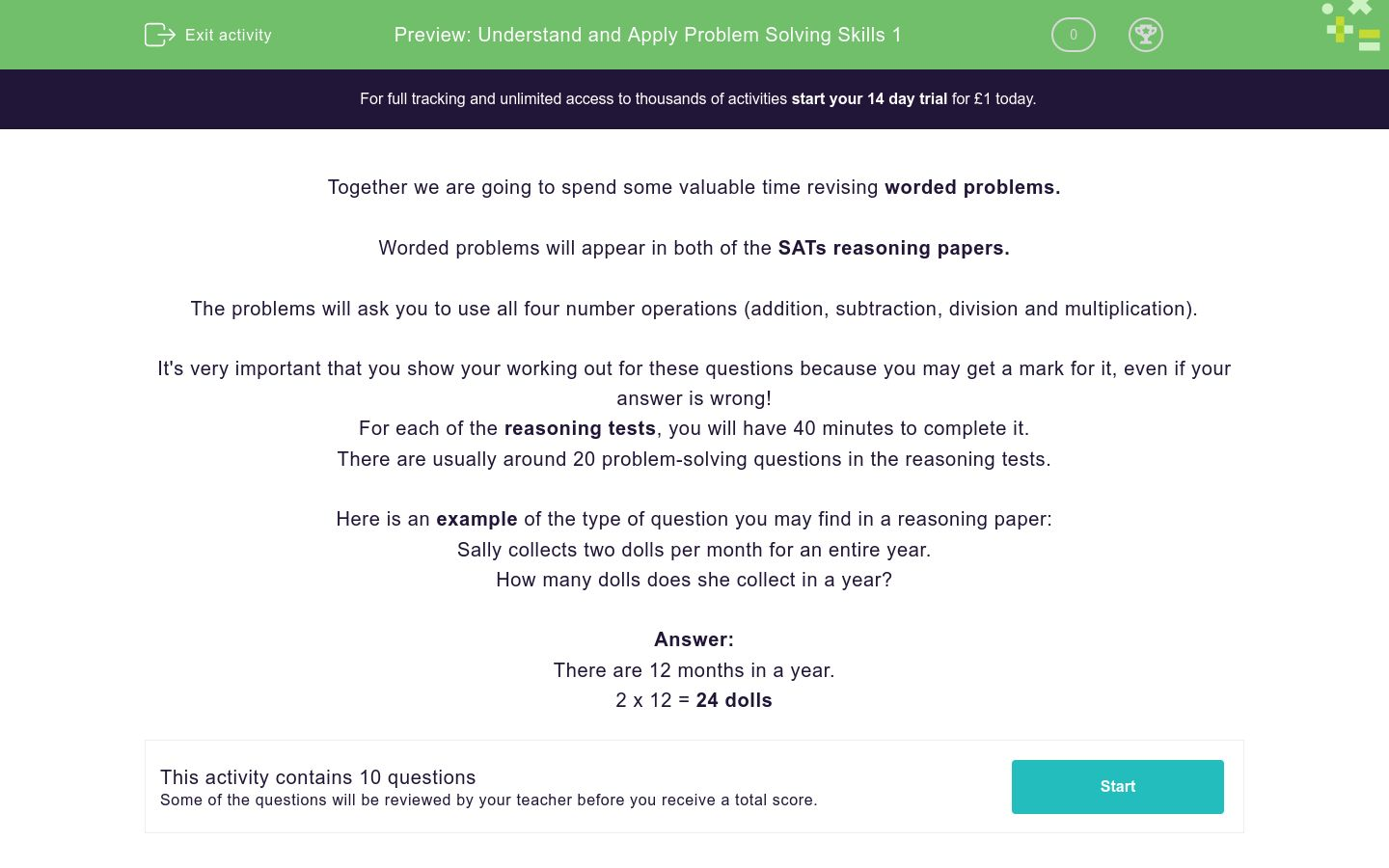# Understand and Apply Problem Solving Skills 1

In this worksheet, students will be asked to solve a range of worded problems, using four operations. These types of problems appear in the two reasoning papers.Key stage:  KS 2

Curriculum topic:   Exam-style Questions: SATs Maths

Curriculum subtopic:   Exam-Style Questions: Worded Problems

Difficulty level:#### Worksheet Overview

Together we are going to spend some valuable time revising worded problems.

Worded problems will appear in both of the SATs reasoning papers.

The problems will ask you to use all four number operations (addition, subtraction, division and multiplication).

It's very important that you show your working out for these questions because you may get a mark for it, even if your answer is wrong!

For each of the reasoning tests, you will have 40 minutes to complete it.

There are usually around 20 problem-solving questions in the reasoning tests.

Here is an example of the type of question you may find in a reasoning paper:

Sally collects two dolls per month for an entire year.

How many dolls does she collect in a year?